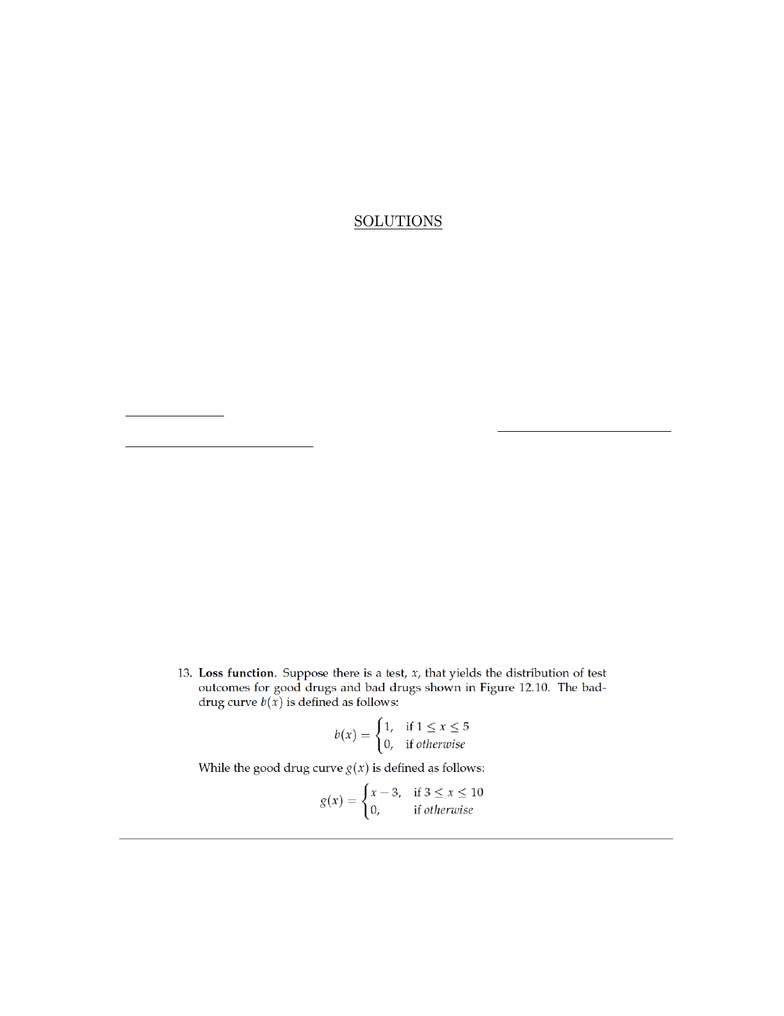Study Guides (380,000)
US (220,000)
Berkeley (5,000)
ECON (200)
Snider (10)
Final

ECON 157 Study Guide - Final Guide: Problem Set, Form 10-Q, LifesavingExam

Department
Economics
Course Code
ECON 157
Professor
Snider
Study Guide
Final

This preview shows pages 1-3. to view the full 15 pages of the document.ECON 157 Health Economics
Problem Set # 3
SOLUTIONS
For this assignment you will clearly answer all parts of the questions given below. The purpose
of this assignment is to explore in depth the material that you have learned in class. Most exer-
cises are quantitative in nature, and are similar in spirit to the questions that will be on your exams.
You can discuss your answers and the problems with others in class, but must turn in your own
solutions. This means that it is ok if your answers are the same as those who you have discussed
the problems with, but that everyone must turn in their own copy of the problem set answers.
Solutions to the problem set will be given immediately after the deadline has passed on midnight
Tuesday, April 29. This is so that everyone in class can have maximum possible time to study
the solutions prior to the ﬁnal on May 14. As a result of this, no late assignments will be
accepted for this problem set. If you dont have the assignment handed in before I send out
the solutions after the due date, you will not get credit for the problem set.
Instructions on how you can hand in the assignment are included at the end of the assignment.
Problems to Answer from Book (2 Problems)
Pharmaceutical Markets
Battacharya Ch. 12, Questions 13-14
1

Only pages 1-3 are available for preview. Some parts have been intentionally blurred.Figure 12.10: Bad drug and good drug distributions for exercise 13.
x
Number of drugs
1
b(x)
g(x)
0 1 3 5 10
(a) Let “total Type I loss” equal the total area under the bad-drug curve to
the right of x, the drug acceptance threshold. In other words, it is the
number of bad drugs approved with this drug acceptance drug. Write
down an equation for total Type I loss as a function of x. The function
L1(x)should indicate how much total Type I loss there is for any value
of x.
Let L1equal total Type I loss. Then:
L1(x)=
4, if x<1
5x, if 1 x<5
0, if 5 x
(b) Now write down an function for total Type II loss as a function of x.
This function L2(x)should give the number of good drugs not ap-
proved given threshold x.
Let L2equal total Type II loss. Then:
L2(x)=
0, if x<3
(x3)2
2, if 3 x<10
24.5, if 10 x
(c) A loss function speciﬁes the welfare loss caused by Type I and Type II
error. If we assume society values each loss type equally, the social loss
function will be the sum of the Type I loss and Type II loss. Calculate
social loss as a function of x.

Only pages 1-3 are available for preview. Some parts have been intentionally blurred.Let Ltotal equal total social loss. Then:
Ltotal(x)=
4, if x<1
5x, if 1 x<3
(x3)2
2+5x, if 3 x<5
(x3)2
2, if 5 x<10
24.5, if 10 x
(d) Draw a graph of your loss function plotted against x. Minimize your
function from the previous exercise to show that the optimal value of x
is 4.
The graph of Ltotal is as follows:
x
Number of drugs
0 1 3 5 10
To ﬁnd x, we take the derivative of the loss function in the region
3<x<5, set it equal to 0, and solve for x:
Ltotal =(x3)2
2+5x
dLtotal
dx =(x3)1
0=(x3)1
x=4.
(e) Explain why the optimal value will always come at the intersection of
the bad drug and good drug distributions if they are both continuous
and overlapping.
6